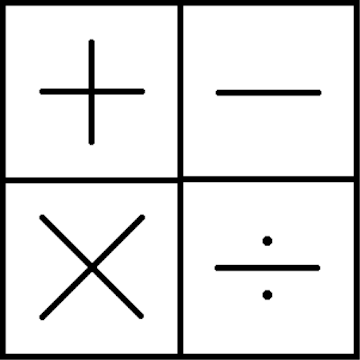# Super Mathobjective of the game to obtain the 3-digit number indicated above, gold color, with 5 moves that consist in the use of one of the 4 operations.
Also, for each move, you can delete a digit from one of the two numbers with which you are doing the operation.

how to play:

at the beginning of the game are indicated:
a basic 3-digit number (green or white)
the number to be reached, always 3 digits in yellow color

for each move:

at the beginning an additional 2-digit number is assigned (operand)

1) you can remove a digit from the operand or from the base number (optional)

2) then you can add or subtract or multiply or divide the base number for the operand:

Subtraction must be: base number minus operand

the division must be: base number divided by the operand

3) the result will be the new base number

the game ends after the fifth move.

score categories:

gold:
if, in any of the 5 rounds, the total is equal to the target number (in this case the game ends)

silver:
if, after the fifth round, the total is close to the destination number
bronze:
if, after the fifth round, the total is less close to the destination number

the range of scoring categories is always shown at the beginning of the game

the target number is shown in gold (yellow)

in online multiplayer, the numbers: target, initial total and all the operands are the same for everyone and the one that comes closest to the target wins